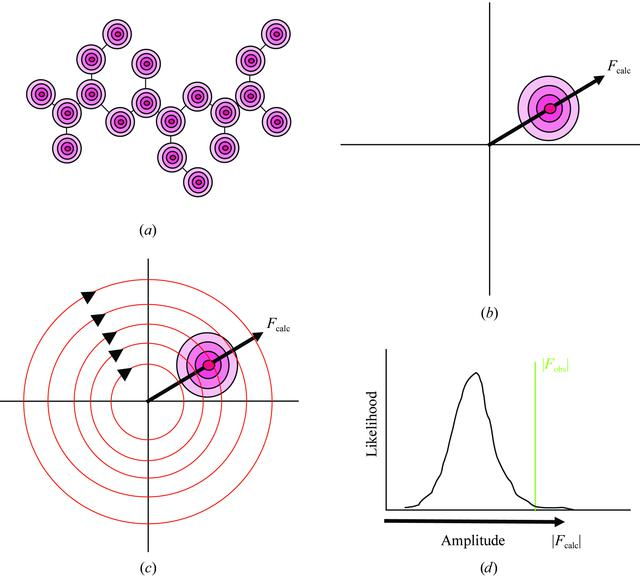disable zoom     view article Figure 2 Probability distributions for one reflection in the maximum-likelihood worldview. (a) The maximum-likelihood method begins with the assumption that the current structural model itself contains errors. This figure represents the probability distributions of the atoms in the model. Instead of a single location, as assumed by the least-squares method, there is a cloud of locations that each atom could occupy. While not required by maximum likelihood, the computer programs available today assume that the distributions of positions are normal and have equal standard deviations [the value of which is defined to be that value which optimizes the fit of the model to the test set of diffraction data (Pannu & Read, 1996; Brünger, 1992)]. (b) The distribution of structures shown in (a) results in a distribution of values for the complex structure factors calculated from that model. An example of one of the distributions is shown. The value of the structure factor calculated from the most probable model is labeled Fcalc. The nonlinear relationship between real and reciprocal space causes this value not to be the most probable value for the structure-factor distribution. As shown by Read (1986), the most probable value has the same phase as Fcalc but has an amplitude that is only a fraction of that of Fcalc. This fraction, conventionally named D, is equal to unity when the model is infinitely precise and is zero when the model is infinitely uncertain. The width of the distribution, named σcalc, also arises from the coordinate uncertainty and is large when D is small and zero when D is unity. The recognition that the structure factor calculated from the most probable model is not the most probable value for the structure factor is the key difference between least squares and the current implementations of maximum likelihood. (c) In refinement without experimental phase information, the probability distribution of the calculated value of the structure factor must be converted to a probability distribution of the amplitude of this structure factor. This transformation is accomplished by mathematically integrating the two-dimensional distribution over all phase angles at each amplitude. This integral is represented by a series of concentric circles. (d) The probability distribution for the amplitude of the structure factor. The bold arrow below the horizontal axis represents the amplitude of Fcalc, calculated from the most probable model. As expected, the most probable amplitude is smaller than |Fcalc|. With this distribution the likelihood of any value for |Fobs| can be evaluated, but more importantly one can calculate how to modify the model to increase the likelihood of |Fobs|. In this example, the likelihood of |Fobs| is improved by either increasing |Fcalc| or increasing the precision of the model. This action is the opposite of the action implied by the least-squares analysis of Fig. 3.BIOLOGICALCRYSTALLOGRAPHY
ISSN: 1399-0047
Volume 60| Part 12| November 2004| Pages 2156-2168